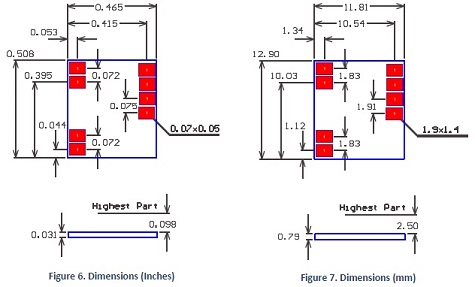# PDu100 Micro Piezo Driver

#### Piezoelectric Drivers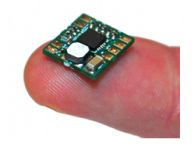### Specifications:

PDu100 Specifications
Power Supply 3 V to 5.5 V
Max. Unipolar Output +100V
Max. Bipolar Output +/-100 V
RMS Output Current 33 mA
Average DC Current 15 mA
Power Bandwidth 3.2 kHz
Peak Output Current 100 mA
Dimensions 11.8 x 12.9 mm (0.46 x 0.51 in)
Weight 560 mg (0.018 oz)
Gain 27.5 V/V
Input Voltage 0.5 Vs +/- 1.8V
Input Offset +/- 100 mV
Noise 70 mV RMS (10uF Load)
Environment 0 to 70 Celsius (-32 to 158 F)
Quiescent Current 25 mA (1 mA in Shutdown)

### Features

The PDu100 is the industry’s smallest and lightest driver for piezoelectric actuators. The PDu100 can drive two-wire piezoelectric actuators and benders up to +/-100 V. The PDu100 can also drive three-wire piezoelectric benders and stack actuators up to +100 V. Applications include battery powered robotics, piezoelectric motors, and ultra low-power positioning and manipulation systems.

The PDu100 is protected against short circuit, current overload, and excessive temperature. A shutdown pin is also provided that reduces the supply current to 1 mA when pulled low. The output voltage range and gain of the PDu100 is customizable to meet the requirements of OEM applications.

### Compatible Actuators

 Stack Actuators up to 100V Plates and Tubes Up to +/-100V Two Wire Benders Up to +/-100V Three Wire Benders 0 to 100V with 100V bias

### Connection Diagram### Operation

The system block diagram is illustrated in Figure 2. A boost converter generates a high-voltage rail to supply a pair of complementary amplifiers. A single output can be used to drive a unipolar load up to +100 V or both amplifiers can be used to produce +/-100 V.

The input is selectable between a unipolar signal biased at half the supply voltage or a bipolar signal. The amplifier gain is 27.5 so a 3.6 Vp-p input will produce a 100 Vp-p output. Both amplifier channels are biased at half the output range (50 V).The overall system gain is determined by the output configuration. The possible combinations are listed below.

System Gain and Voltage Range
Output TypeGainInput RangeOutput Range
Unipolar 27.5 0.5 Vs +/- 1.8V 100V
Bipolar 55 0.5 Vs +/- 1.8V +/- 100V

Both outputs are biased at approximately half the HV bus voltage, e.g. 50 V. The output voltage equations are listed below.

System Gain and Voltage Range
Output TypeOutput Equation
Unipolar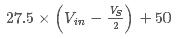Bipolar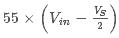The gain and output voltage ranges can be customized by contacting Micromechatronics.

### Example Applications

Some typical application circuits are shown below. The optional output resistance Ro is used to reduce noise as described in “Noise”. For general purpose applications, the recommended value for Ro is 270 Ohms.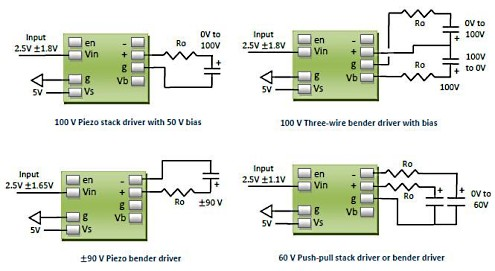### Power Bandwidth

The power bandwidth is the maximum frequency periodic signal that can be reproduced without distortion. The power bandwidth calculator determines the maximum operating frequency and required power for a given load capacitance. The calculator includes the effects of current limit, slew-rate, and signal bandwidth.

The calculator does not include the time-constant of the peak current limit, and therefore may become inaccurate when the power bandwidth is below 50 Hz.Click Icon to open

### Noise

The output voltage of the PDu100 contains switching noise from the boost converter and random noise from the high-voltage amplifier. The amount of noise seen by the load capacitance is determined by the size of the output resistance and signal bandwidth. If there is no output resistance, a value of 100 Ohms can be used to calculate the bandwidth and predict noise.

To determine the output resistance required for a particular noise level, the required bandwidth should be selected from Figure 9 below. The correct resistance can then be calculated from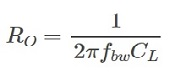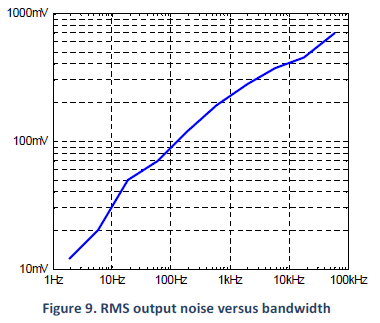The noise measurements are performed with a static input voltage. When current is drawn from the output, the ripple will increase due to action from the boost converter.

### Signal Bandwidth

The unloaded small signal bandwidth of the PDu100 is approximately 60 kHz. With a capacitive load, the signal bandwidth is determined by the output resistance, that is### Supply Current

The supply current IS is related to the load current IL through the following power balance equationwhere VS is the supply voltage. With a capacitive load and sinusoidal voltage, the peak and average output current is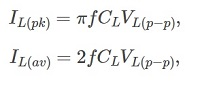where VL is the peak to peak voltage across the load capacitance. The average supply current can be written### Power Dissapation

With a capacitive load, power dissipation is the product of supply voltage and the average current, that is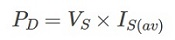When operating at full power bandwidth, the worst-case power dissipation is approximately 2.5 W. The thermal impedance of the PDu100 from junction to ambient is 45 K/W . Therefore, the maximum temperature rise is approximately 90 degrees C above ambient. When continuous power dissipation above 1 W is required, the PDu100 is designed to be mounted onto a thermal sink using a thermally conductive double-sided adhesive such as 3M 8940 or Bergquist BOND-PLY 100.

### Enable / Shutdown

The Enable pin can be pulled low to disable the amplifier and reduce the quiescent current to 1 mA. It can be driven by a logic output or an open collector output. The recovery time after a shut-down is 2 ms.

The PDu100 is protected against over-current and thermal overload. If the temperature exceeds 150 degrees C, the amplifier will be disabled until the temperature reduces.

### Dimensions